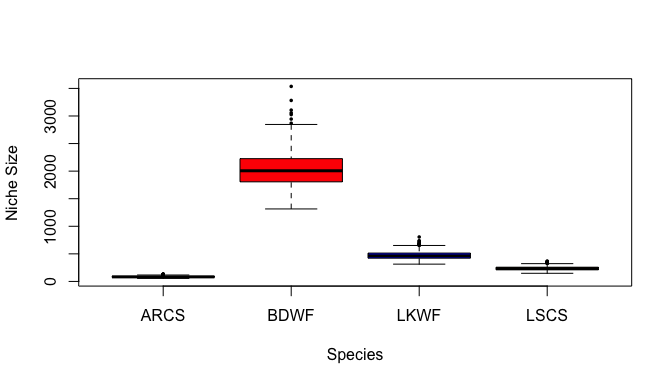# An Ecologist’s Guide to nicheROVER: NicheRegion and Niche Overlap Metrics for Multidimensional Ecological Niches

### 1. Preliminaries

For the beginner or first-time user, you will need to familiarize yourself with the following functions and help pages: niche.plot(), niche.size(), overlap(), and overlap.plot().

### 2. Formatting data for use in nicheROVER

The first step is to format the data so that it can be properly read into functions. This should be in a data.frame set up, with niche variables (e.g., stable isotope, habitat or contaminant variables - stable isotope in this case) along the columns and observations along the rows. This vignette will use the example dataset fish in the nicheROVER package, which contains the stable isotope values of $$\delta^{15}\textrm{N}$$, $$\delta^{13}\textrm{C}$$, and $$\delta^{34}\textrm{S}$$ from muscle tissue for 278 individual fish belonging to four arctic fish species (see ?fish for more information on the sample dataset).

We import the data using the data() function. We then calculate the means for each isotope and species using the aggregate() function:

# analysis for fish data

library(nicheROVER)
data(fish) # 4 fish, 3 isotopes
aggregate(fish[2:4], fish, mean) # isotope means calculated for each species
##   species      D15N      D13C      D34S
## 1    ARCS 12.609420 -23.96812 14.565652
## 2    BDWF  9.270282 -26.70352 -3.149437
## 3    LKWF 11.036418 -25.15299  6.101493
## 4    LSCS 11.721000 -25.15471 11.451429

### 3. Generate the posterior distributions of $$\mu$$ (mean) and $$\Sigma$$ (variance) for isotope values for each species with the default prior

This step is not absolutely necessary in generating the niche region and overlap plots, but can be useful during exploratory data analyses.

# fish data
data(fish)

# generate parameter draws from the "default" posteriors of each fish
nsamples <- 1e3
system.time({
fish.par <- tapply(1:nrow(fish), fish$species, function(ii) niw.post(nsamples = nsamples, X = fish[ii,2:4])) }) ## user system elapsed ## 0.143 0.017 0.180 # various parameter plots clrs <- c("black", "red", "blue", "orange") # colors for each species # mu1 (del15N), mu2 (del13C), and Sigma12 par(mar = c(4, 4, .5, .1)+.1, mfrow = c(1,3)) niche.par.plot(fish.par, col = clrs, plot.index = 1) niche.par.plot(fish.par, col = clrs, plot.index = 2) niche.par.plot(fish.par, col = clrs, plot.index = 1:2) legend("topleft", legend = names(fish.par), fill = clrs)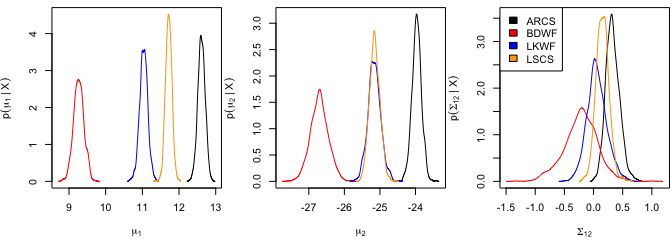# all mu (del15N, del13C, del34S) niche.par.plot(fish.par, col = clrs, plot.mu = TRUE, plot.Sigma = FALSE) legend("topleft", legend = names(fish.par), fill = clrs)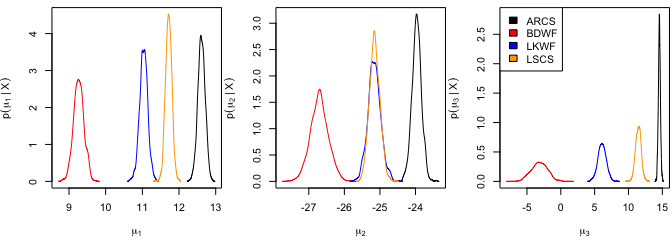# all mu and Sigma par(mar = c(4.2, 4.2, 2, 1)+.1) niche.par.plot(fish.par, col = clrs, plot.mu = TRUE, plot.Sigma = TRUE) legend("topright", legend = names(fish.par), fill = clrs)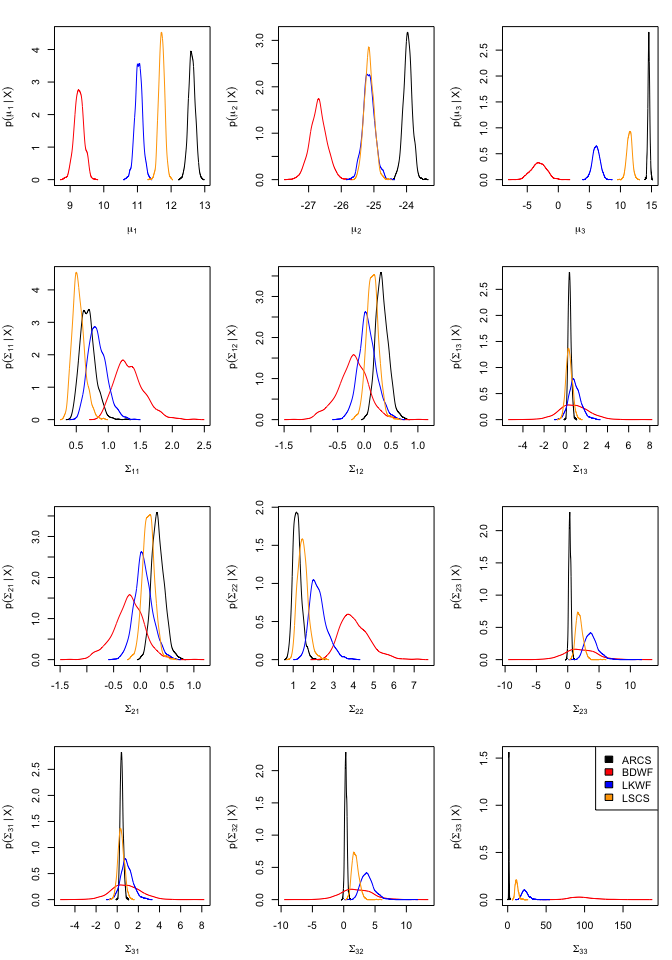### 4. Create 2-d projections of a subset of niche regions See ?niche.plot for more details on parameter values. Here, we have chosen to display 10 random niche regions generated by the Bayesian analysis. The parameter list fish.par is generated using the niw.post() function provided by nicheROVER. The resulting figure generates niche plots, density distributions, and raw data for each pairwise combination of isotope data for all four fish species (i.e., bivariate projections of 3-dimensional isotope data). # 2-d projections of 10 niche regions clrs <- c("black", "red", "blue", "orange") # colors for each species nsamples <- 10 fish.par <- tapply(1:nrow(fish), fish$species,
function(ii) niw.post(nsamples = nsamples, X = fish[ii,2:4]))

# format data for plotting function
fish.data <- tapply(1:nrow(fish), fish$species, function(ii) X = fish[ii,2:4]) niche.plot(niche.par = fish.par, niche.data = fish.data, pfrac = .05, iso.names = expression(delta^{15}*N, delta^{13}*C, delta^{34}*S), col = clrs, xlab = expression("Isotope Ratio (per mil)"))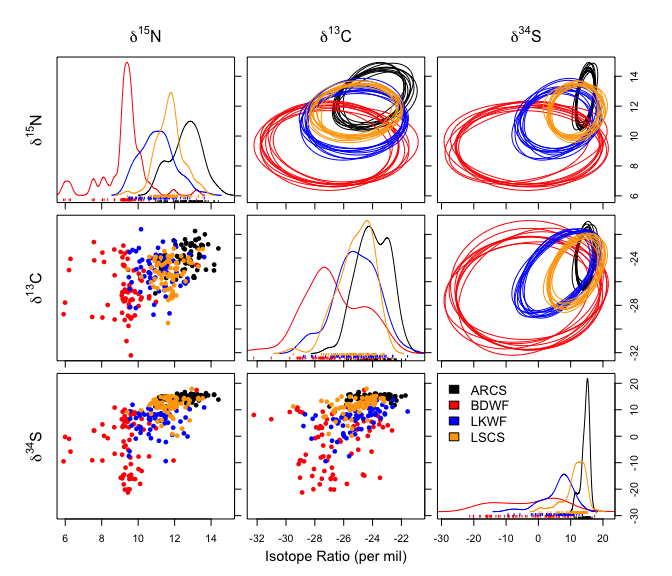### 5. Calculate and display niche overlap estimates We use the function overlap() to calculate overlap metric estimates from a specified number of Monte Carlo draws (nsamples) from the fish.par parameter list. It is necessary to specify the $$\alpha$$-level. In this case, we have decided to calculate the overlap metric at two niche regions sizes for comparison: alpha=0.95 and alpha=0.99, or 95% and 99%. Then, we calculate the mean overlap metric between each species. Remember that the overlap metric is directional, such that it represents the probability that an individual from Species $$A$$ will be found in the niche of Species $$B$$. # niche overlap plots for 95% niche region sizes nsamples <- 1000 fish.par <- tapply(1:nrow(fish), fish$species,
function(ii) niw.post(nsamples = nsamples, X = fish[ii,2:4]))

# Overlap calculation.  use nsamples = nprob = 10000 (1e4) for higher accuracy.
# the variable over.stat can be supplied directly to the overlap.plot function

over.stat <- overlap(fish.par, nreps = nsamples, nprob = 1e3, alpha = c(.95, 0.99))

#The mean overlap metrics calculated across iteratations for both niche
#region sizes (alpha = .95 and alpha = .99) can be calculated and displayed in an array.
over.mean <- apply(over.stat, c(1:2,4), mean)*100
round(over.mean, 2)
## , , alpha = 95%
##
##          Species B
## Species A  ARCS  BDWF  LKWF  LSCS
##      ARCS    NA 11.25 66.21 81.63
##      BDWF  0.31    NA 25.96  4.52
##      LKWF  7.33 78.17    NA 52.86
##      LSCS 37.58 51.79 88.55    NA
##
## , , alpha = 99%
##
##          Species B
## Species A  ARCS  BDWF  LKWF  LSCS
##      ARCS    NA 33.32 87.29 92.08
##      BDWF  0.79    NA 41.46  8.94
##      LKWF 11.67 92.19    NA 70.23
##      LSCS 50.05 80.14 97.05    NA
over.cred <- apply(over.stat*100, c(1:2, 4), quantile, prob = c(.025, .975), na.rm = TRUE)
round(over.cred[,,,1]) # display alpha = .95 niche region
## , , Species B = ARCS
##
##        Species A
##         ARCS BDWF LKWF LSCS
##   2.5%    NA    0    4   28
##   97.5%   NA    1   12   49
##
## , , Species B = BDWF
##
##        Species A
##         ARCS BDWF LKWF LSCS
##   2.5%     1   NA   59   24
##   97.5%   32   NA   93   82
##
## , , Species B = LKWF
##
##        Species A
##         ARCS BDWF LKWF LSCS
##   2.5%    43   16   NA   77
##   97.5%   89   37   NA   97
##
## , , Species B = LSCS
##
##        Species A
##         ARCS BDWF LKWF LSCS
##   2.5%    66    2   39   NA
##   97.5%   92    8   67   NA

In the returned plot, Species $$A$$ is along the rows and Species $$B$$ is along columns. The plots represent the posterior probability that an individual from the species indicated by the row will be found within the niche of the species indicated by the column header. Before you plot, you must decide upon your $$\alpha$$-level, and make sure the variable over.stat reflects this choice of $$\alpha$$.

# Overlap plot.Before you run this, make sure that you have chosen your
#alpha level.
clrs <- c("black", "red", "blue", "orange") # colors for each species
over.stat <- overlap(fish.par, nreps = nsamples, nprob = 1e3, alpha = .95)
overlap.plot(over.stat, col = clrs, mean.cred.col = "turquoise", equal.axis = TRUE,
xlab = "Overlap Probability (%) -- Niche Region Size: 95%")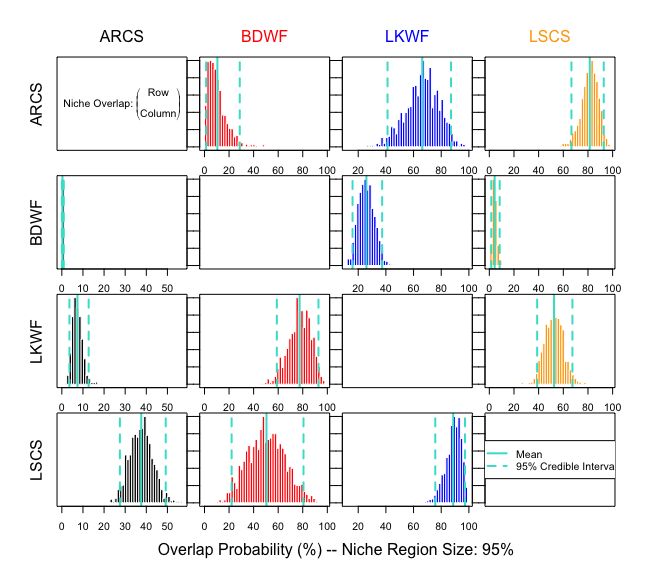### 6. Calculate and display niche size estimates

See ?niche.size for exactly how niche size is defined as a function of the parameters $$\mu$$ and $$\Sigma$$. In a Bayesian context, we calculate the posterior distribution of niche size by species. This done by calculating the niche size for every posterior sample of $$\mu$$ and $$\Sigma$$.

# posterior distribution of (mu, Sigma) for each species
nsamples <- 1000
fish.par <- tapply(1:nrow(fish), fish$species, function(ii) niw.post(nsamples = nsamples, X = fish[ii,2:4])) # posterior distribution of niche size by species fish.size <- sapply(fish.par, function(spec) { apply(spec$Sigma, 3, niche.size, alpha = .95)
})

# point estimate and standard error
rbind(est = colMeans(fish.size),
se = apply(fish.size, 2, sd))
##         ARCS      BDWF      LKWF      LSCS
## est 84.33841 2026.8192 470.74044 236.57088
## se  12.75944  301.5408  71.25809  34.43926
# boxplots
clrs <- c("black", "red", "blue", "orange") # colors for each species
boxplot(fish.size, col = clrs, pch = 16, cex = .5,
ylab = "Niche Size", xlab = "Species")